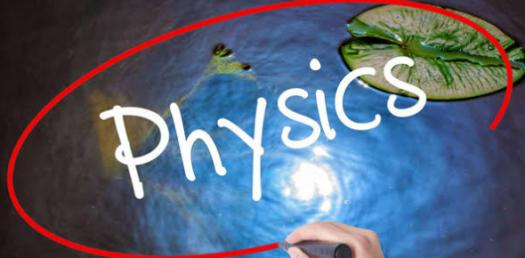# Trivia Questions Quiz: Can You Pass This Toughest Physics Test?

38 Questions | Total Attempts: 48SettingsCan you pass this toughest physics test? A lot of people take up physics and get a negative review of it and see it as one of the hardest subject one can take. How about you take up the interesting quiz below and get to refresh your understanding on the major topics you might be tested in the exams? All the best!

• 1.
______ : Has magnitude only
• 2.
______ : The most fundamental of the physical sciences
• 3.
______ : Displacement/travel time
• 4.
______ : Velocity at an instand of time
• 5.
______ : Directed toward the center of a circular motion
• 6.
______ : Actual path length
• 7.
______ : Straight-line directed distance
• 8.
______ : A continuous change of position
• 9.
______ : Time rate of change of velocity
• 10.
______ : Has magnitude and direction
• 11.
______ : Motion soley under the influence of gravity
• 12.
______ : Motion of a thrown object
• 13.
______ : Speed at an instant of time
• 14.
______ : The location of an object
• 15.
______ : 9.8 m/s^2
• 16.
______ : Distance traveled/travel time
• 17.
______ : Differences between final and initial velocities divided by time
• 18.
______ : Zero acceleration in free-fall
• 19.
What is necessary to designate a position?
• A.

Fundamental units

• B.

Motion

• C.

A direction

• D.

A reference point

• 20.
Which one of the following describes an object in motion?
• A.

A period of time has passed.

• B.

Its position is known.

• C.

It is continuously changing position.

• D.

It has reached its final position.

• 21.
Unlike scalars, vectors have which of the following?
• A.

Magnitude

• B.

Units

• C.

Position

• D.

Direction

• 22.
Which one of the following does NOT have units of m/s?
• A.

Average speed

• B.

Average acceleration

• C.

Average velocity

• D.

Terminal velocity

• 23.
For a constant linear acceleration, what changes uniformly?
• A.

Acceleration

• B.

Velocity

• C.

Distance

• D.

Displacement

• 24.
Which one of the following is true for a deceleration?
• A.

The velocity remains constant.

• B.

The acceleration is negative.

• C.

The acceleration is in the direction opposite to the velocity.

• D.

The acceleration is zero.

• 25.
If the speed of an onject in uniform circular motion is doubled and the radial distance remains constant, then the magnitude of the centripetal acceleration increases by what factor?
• A.

2

• B.

3

• C.

4

• D.

6

Related TopicsBack to top